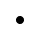Mobile4August 5, 2022

# Artificial intelligence algorithms #3 - Simulated annealing

Simulated Annealing is inspired by annealing in metallurgy. It consists in heating the metal to a high temperature and then slowly cooling it. In this way, the internal structure of the metal is first broken and then very slowly "repaired". This results in a metal that has significantly better properties than at the beginning of the process.

Very simple video showing annealing in metallurgy / jewelry making 👇

Annealing in metallurgy can also take this form

Remember how at HillClimbing and TabuSearch we looked at all the neighbors and picked the best one? Simulated annealing does the same thing, but... But with some probability, it is willing to accept a worse solution. If we decide on a worse solution at the right moment, we can later get to a better one = get out of the local minimum/maximum.

## Use case

Before we dive into the algorithm, I will briefly consider when simulated annealing is used... It is an ideal candidate if we have to optimize several parameters of the same type at once (vectors). A classic example is the problem of a business traveler, or the optimization of fuel consumption on a road. If you want to try this algorithm, I again recommend codingame.com

I personally use this algorithm when I want to reach at least a satisfactory solution extremely quickly. If I want the solution to be as accurate as possible, I turn to genetic algorithms, which we will cover later.

## Algorithm

As with other optimization algorithms, we will create a random solution. However, its optimization will consist in lowering the temperature a pre-defined number of times (n) and based on this temperature and the generated neighbor we can accept or reject it. A simplified form of the algorithm would work like this:

1. solution = random solution
2. temperature = initial temperature
3. n times
a. neighbor_solution = neighborhood solution based on solutions
b. sneighboring_solution = is better than solution

i.  true ⇒ solution = neighbor_solution

ii. false ⇒solution =  neighbor_solution if temperature is high enough???

c. lower temperature

4. Return best solution found yet

### When is the temperature high enough?

Kedy je však teplota dosť vysoká aby sme susedne_riesenie akceptovali (bod 3b)? Sem prichádza na rad klasický vzorec, ktorý nám pozorovanie žíhania prinieslo. susedne_riesenie akceptujeme s pravdepodobnosťou:

But when is the temperature high enough for us to accept the neighbor_solution (point 3b)? This is where the classic formula that the observation of annealing brought us comes into play. We accept neighbor_solution with probability:

P(∆E)=e^{\frac{-∆E}{t}}

Where ∆E is the score difference of the neighboring solution and the original solution. Although the formula looks a little scary, the implementation of this formula is actually simple.

### How do we lower the temperature?

We can reduce the temperature in different ways (point 3c). You can probably think of several ways, but let's write them down:

1. Linear temperature reduction: T' = T - α
2. Geometric temperature reduction: T' = T . α
3. Slow temperature reduction: T' = T/(1+βT)

## Variants

When I studied this algorithm, it became clear that many people have a different idea of its use. Basically there are 2 main use cases:

1. We get a neighbor with a small change and accept him with a certain probability.
2. The higher the temperature, the more significant changes we can make in the neighbor.

Furthermore, the algorithm can vary, for example, in when we change the temperature. Someone changes the temperature in each iteration, someone lets the algorithm cool down every n-iterations.

No one can simply say how to set the initial temperature, and no one can say how fast to let the temperature cool down (value of α).

## Sulution for Eight queens puzzle

Let's see again how the eight queens problem can be solved in that case. If you are not already familiar with this issue, see the article dedicated to TabuSearch.

1. We create a random solution (1).

2. We start the main cycle (3) n times.

3. in the cycle we get a new temperature (3c). Although I wrote about gradually lowering the temperature, I can also get a lower and lower temperature this way:

4. we get a new neighbor_solution (3a). In our case, I will move one random queen to a random row in the same column.

5. if we pass the acceptance function (3b), we save the new neighbor as the current solution.

You can find the entire program on GitHub

Unlike TabuSearch, simulated annealing found a solution for 20 queens and even found it in 30 milliseconds, for 40 queens in 40-200 ms. For 50 checkers I managed to find a solution in 200-800 ms. The biggest chessboard I solved was 80x80 (~10s). Such results are unimaginable with previous algorithms.

Solution "8, 13, 3, 17, 7, 10, 4, 0, 18, 15, 6, 11, 19, 12, 16, 2, 5, 1, 9, 14" found in 3028 iterations and 30ms

### A solution to the business traveler's problem

I have already mentioned the problem of the business traveler. This problem deserves a separate article, so I will not cover it here. Using simulated annealing, my solution looks like this.

For 50 cities, simulated annealing finds a satisfactory solution within a few seconds.

❗️ Attention! The video is significantly slowed down 🙂

So what? Are you starting to feel like we're getting closer to a little smarter programs? The Genetic Algorithm awaits us next time. One of my favorites!